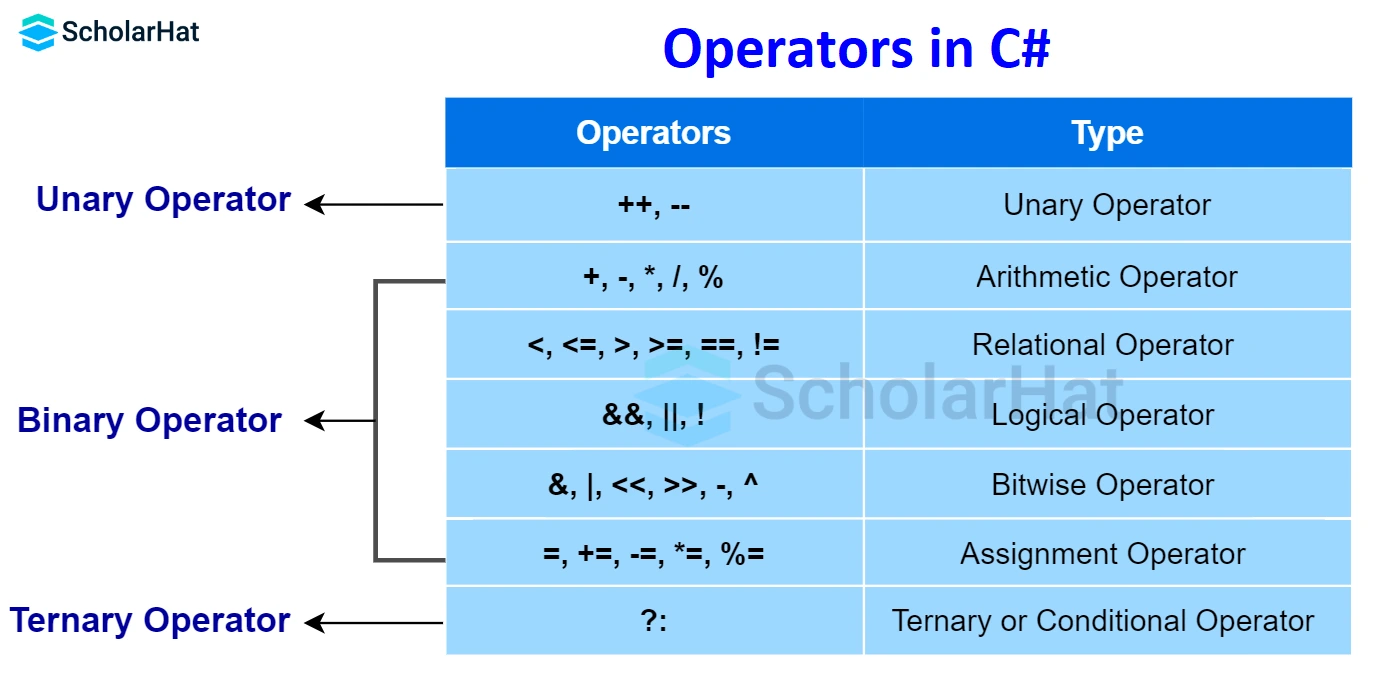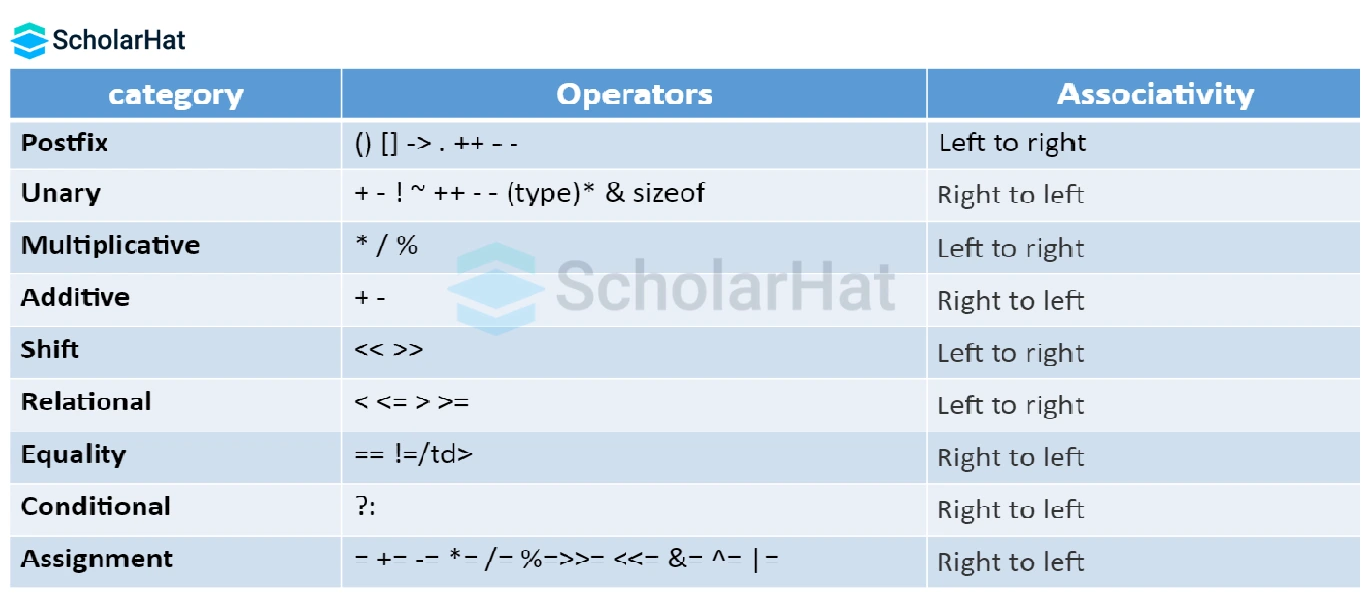# Operators in C#: Arithmetic, Comparison, Logical and More...

17 Nov 2023
Beginner
166 ViewsLearn via Video Course & by Doing Hands-on Labs

## Operators in C#: An Overview

Operators play a crucial role in any Programming Language, including C#. They allow us to perform various operations on data types, making our code more efficient and concise. In this article, we will explore the different types of Operators in C# and understand how they can enhance our coding efficiency.

## Different Types of Operators in C#

C# provides a wide range of operators that cater to different requirements. Let's take a look at the different types of operators in C#:### Arithmetic Operators

Arithmetic operators are used to perform mathematical calculations in C#. They include addition (+), subtraction (-), multiplication (*), division (/), and modulus (%). These operators allow us to manipulate numerical values and perform calculations with ease. For example, if we want to add two numbers together, we can simply use the addition operator (+).

#### Explanation

This C# code defines a console application that performs basic arithmetic operations. It initializes two integers (x=10 and y=5), then calculates and prints the results of addition, subtraction, multiplication, division, and modulo operations between these two numbers using appropriate operators.

#### Output

``````Addition Operator: 15
Subtraction Operator: 5
Multiplication Operator: 50
Division Operator: 2
Modulo Operator: 0``````

### Assignment Operators

Assignment operators are used to assign values to variables in C#. They include the simple assignment operator (=), as well as compound assignment operators such as +=, -=, *=, and /=. These operators not only assign values but also perform an operation simultaneously. For instance, the += operator adds the right-hand operand to the left-hand operand and assigns the result to the left-hand operand.

#### Assignment Operators: Example

``` `using System;````namespace Assignment {

class DNT {
// Main Function
static void Main(string[] args)
{
// initialize variable x
// using Simple Assignment
// Operator "="
int x = 15;
// it means x = x + 10
x += 10;
Console.WriteLine("Add Assignment Operator: " + x);
// initialize variable x again
x = 20;
// it means x = x - 5
x -= 5;
Console.WriteLine("Subtract Assignment Operator: " + x);
// initialize variable x again
x = 15;
// it means x = x * 5
x *= 5;
Console.WriteLine("Multiply Assignment Operator: " + x);
// initialize variable x again
x = 25;
// it means x = x / 5
x /= 5;
Console.WriteLine("Division Assignment Operator: " + x);
// initialize variable x again
x = 25;
// it means x = x % 5
x %= 5;
Console.WriteLine("Modulo Assignment Operator: " + x);
// initialize variable x again
x = 8;
// it means x = x << 2
x <<= 2;
Console.WriteLine("Left Shift Assignment Operator: " + x);
// initialize variable x again
x = 8;
// it means x = x >> 2
x >>= 2;
Console.WriteLine("Right Shift Assignment Operator: " + x);
// initialize variable x again
x = 12;
// it means x = x >> 4
x &= 4;
Console.WriteLine("Bitwise AND Assignment Operator: " + x);
// initialize variable x again
x = 12;
// it means x = x >> 4
x ^= 4;
Console.WriteLine("Bitwise Exclusive OR Assignment Operator: " + x);
// initialize variable x again
x = 12;
// it means x = x >> 4
x |= 4;
Console.WriteLine("Bitwise Inclusive OR Assignment Operator: " + x);
}
}
}```
```

#### Explanation

This C# code demonstrates various assignment operators and their usage. It initializes an integer variable "x" and then performs operations like addition, subtraction, multiplication, division, modulo, left shift, right shift, bitwise AND, bitwise XOR, and bitwise OR using their corresponding assignment operators, modifying and printing the value of "x" at each step.

#### Output

``````Add Assignment Operator: 25
Subtract Assignment Operator: 15
Multiply Assignment Operator: 75
Division Assignment Operator: 5
Modulo Assignment Operator: 0
Left Shift Assignment Operator: 32
Right Shift Assignment Operator: 2
Bitwise AND Assignment Operator: 4
Bitwise Exclusive OR Assignment Operator: 8
Bitwise Inclusive OR Assignment Operator: 12``````

### Comparison Operators

Comparison operators are used to compare values in C#. They include == (equality), != (inequality), > (greater than), < (less than), >= (greater than or equal to), and <= (less than or equal to). These operators return a Boolean value (true or false) based on the comparison result. For example, if we want to check if two numbers are equal, we can use the equality operator (==).

#### Explanation

This C# program compares two integer variables, num1 and num2, using relational operators. It checks if num1 is equal to num2, not equal, greater than, less than, greater than or equal to, and less than or equal to num2. The results of these comparisons are printed on the console.

#### Output

``````10 == 5 : False
10 != 5 : True
10 > 5 : True
10 < 5 : False
10 >= 5 : True
10 <= 5 : False``````

Logical Operators

Logical operators are used to perform logical operations in C#. They include && (logical AND), || (logical OR), and ! (logical NOT). These operators are commonly used in conditional statements and loops. They allow us to combine multiple conditions and control the flow of our program based on the result. For instance, if we want to check if both condition A and condition B are true, we can use the logical AND operator (&&).

#### Logical Operators: Example

``` ```using System;
namespace Logical {
class DNT {
// Main Function
static void Main(string[] args)
{
bool a = true,b = false, result;
// AND operator
result = a && b;
Console.WriteLine("AND Operator: " + result);
// OR operator
result = a || b;
Console.WriteLine("OR Operator: " + result);
// NOT operator
result = !a;
Console.WriteLine("NOT Operator: " + result);
}
}
}```
```

#### Explanation

This C# code demonstrates the usage of logical operators. It initializes two boolean variables, 'a' and 'b', and then computes and prints the results of logical AND, OR, and NOT operations between these variables. The code shows how these operators combine and negate boolean values to produce logical results.

#### Output

``````AND Operator: False
OR Operator: True
NOT Operator: False``````

### Bitwise Operators

Bitwise operators are used to perform operations at the bit level in C#. They include &, |, ^, ~, <<, and >>. These operators manipulate individual bits of an operand, allowing us to perform operations such as bitwise AND, bitwise OR, bitwise XOR, bitwise complement, left shift, and right shift. Bitwise operators are particularly useful in scenarios where we need to work with binary data or perform low-level operations.

### Unary Operators

Unary operators are used to perform operations on a single operand in C#. They include ++ (increment), -- (decrement), + (positive), - (negative), ! (logical NOT), and ~ (bitwise complement). These operators allow us to modify the value of a variable or change its state based on specific conditions. For example, the increment operator (++) increases the value of a variable by 1.

#### Unary Operators: Example

``` `using System;````namespace Arithmetic {
class DNT {
static void Main(string[] args)
{
int a = 10, res;
res = a++;
Console.WriteLine("a is {0} and res is {1}", a, res);
res = a--;
Console.WriteLine("a is {0} and res is {1}", a, res);
res = ++a;
Console.WriteLine("a is {0} and res is {1}", a, res);
res = --a;
Console.WriteLine("a is {0} and res is {1}",a, res);
}
}
}```
```

#### Explanation

This C# code illustrates the post-increment (a++) and post-decrement (a--) operators, as well as the pre-increment (++a) and pre-decrement (--a) operators. It initializes an integer 'a', performs these operations, and prints 'a' and the result of each operation to demonstrate how these operators affect the variable's value.

#### Output

``````a is 11 and res is 10
a is 10 and res is 11
a is 11 and res is 11
a is 10 and res is 10``````

### Ternary Operators

Ternary operators are unique to C# and allow us to write concise conditional expressions. The ternary operator (?:) takes three operands: a condition, a true expression, and a false expression. It evaluates the condition and returns the true expression if the condition is true, otherwise, it returns the false expression. Ternary operators are handy when we need to assign a value to a variable based on a condition in a single line of code.

#### Ternary Operators: Example

``` ```using System;
class Program
{
static void Main()
{
int number = 10;
// Ternary operator to check if number is even or odd
string evenOrOdd = (number % 2 == 0) ? "Even" : "Odd";
Console.WriteLine(\$"The number {number} is {evenOrOdd}.");
// Ternary operator to find the maximum of two numbers
int a = 5, b = 8;
int max = (a > b) ? a : b;
Console.WriteLine(\$"The maximum of {a} and {b} is {max}.");
// Ternary operator to determine if a person can vote
int age = 17;
string canVote = (age >= 18) ? "Yes" : "No";
Console.WriteLine(\$"Can the person, who is {age} years old, vote? {canVote}.");
}
}```
```

#### Explanation

This C# program uses ternary operators to perform conditional operations in a concise way. It checks if 'number' is even or odd, finds the maximum of 'a' and 'b', and determines if a person can vote based on their 'age'. The results are then printed to the console. Ternary operators provide a compact way to write conditional expressions with a true/false outcome.

#### Output

``````The number 10 is Even.
The maximum of 5 and 8 is 8.
Can the person, who is 17 years old, vote? No``````

## Precedence of Operators in C#

Operators in C# have different levels of precedence, which determines the order in which they are evaluated. It is essential to understand the precedence rules to avoid unexpected results in our code. For example, multiplication (*) has higher precedence than addition (+), so an expression like 2 + 3 * 4 will evaluate to 14, not 20. By understanding the precedence of operators, we can write code that accurately reflects our intended calculations.

Here is the Precedence of Operators in C###### Conclusion

In conclusion, operators are a fundamental aspect of C# programming. They allow us to perform various operations on various data types, enhancing our coding efficiency. By understanding the different types of operators in C#, their functionality, and the precedence rules, we can write code that is concise, efficient, and easy to understand. So, next time you write code in C#, remember to leverage the power of operators and take your coding efficiency to new heights!

## Resources for further learning and practice

Online tutorials and courses: Explore Additional Learning and Practice Resources with us at https://www.scholarhat.com/training/csharp-certification-training

There are numerous online C# tutorials and courses available that specifically focus on C# language.

## Take our free csharp skill challenge to evaluate your skill

In less than 5 minutes, with our skill challenge, you can identify your knowledge gaps and strengths in a given skill.

Share Article
Similar Articles